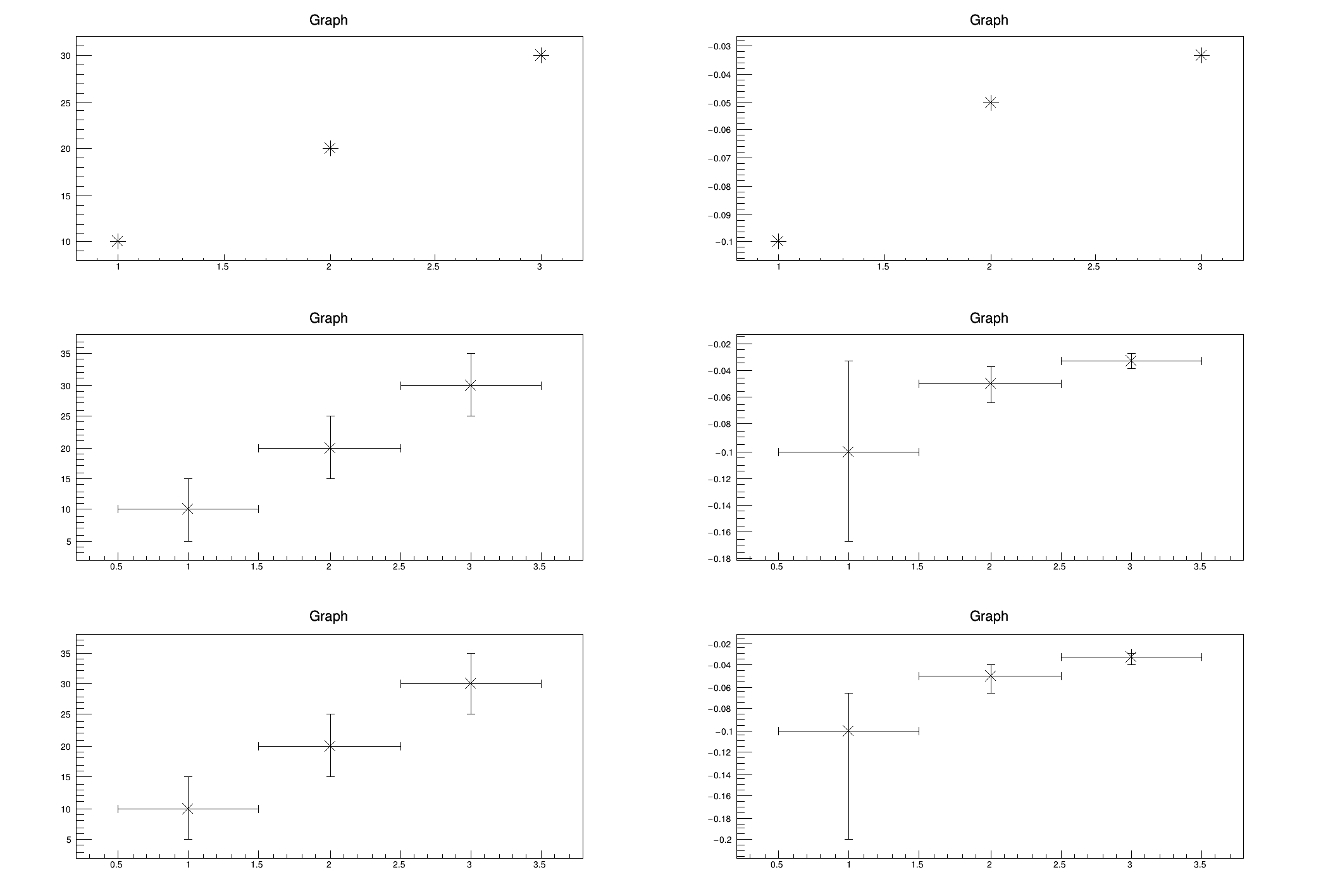ROOT   Reference GuidegraphApply.C File Reference

## Detailed DescriptionA macro to demonstrate the functionality of TGraph::Apply() method.

TGraph::Apply applies a function f to all the data TGraph points. f may be a 1-D function TF1 or 2-d function TF2. The Y values of the graph are replaced by the new values computed using the function.void graphApply()
{
const Int_t npoints=3;
Double_t xaxis[npoints] = {1.,2.,3.};
Double_t yaxis[npoints] = {10.,20.,30.};
Double_t errorx[npoints] = {0.5,0.5,0.5};
Double_t errory[npoints] = {5.,5.,5.};
Double_t exl[npoints] = {0.5,0.5,0.5};
Double_t exh[npoints] = {0.5,0.5,0.5};
Double_t eyl[npoints] = {5.,5.,5.};
Double_t eyh[npoints] = {5.,5.,5.};
TGraph *gr1 = new TGraph(npoints,xaxis,yaxis);
TGraphErrors *gr2 = new TGraphErrors(npoints,xaxis,yaxis,errorx,errory);
TGraphAsymmErrors *gr3 = new TGraphAsymmErrors(npoints,xaxis,yaxis,exl,exh,eyl,eyh);
TF2 *ff = new TF2("ff","-1./y");
TCanvas *c1 = new TCanvas("c1","c1");
c1->Divide(2,3);
// TGraph
c1->cd(1);
gr1->DrawClone("A*");
c1->cd(2);
gr1->Apply(ff);
gr1->Draw("A*");
// TGraphErrors
c1->cd(3);
gr2->DrawClone("A*");
c1->cd(4);
gr2->Apply(ff);
gr2->Draw("A*");
// TGraphAsymmErrors
c1->cd(5);
gr3->DrawClone("A*");
c1->cd(6);
gr3->Apply(ff);
gr3->Draw("A*");
}
int Int_t
Definition: RtypesCore.h:45
double Double_t
Definition: RtypesCore.h:59
The Canvas class.
Definition: TCanvas.h:23
A 2-Dim function with parameters.
Definition: TF2.h:29
TGraph with asymmetric error bars.
virtual void Apply(TF1 *f)
Apply a function to all data points y = f(x,y)
A TGraphErrors is a TGraph with error bars.
Definition: TGraphErrors.h:26
virtual void Apply(TF1 *f)
apply function to all the data points y = f(x,y)
A TGraph is an object made of two arrays X and Y with npoints each.
Definition: TGraph.h:41
virtual void Draw(Option_t *chopt="")
Draw this graph with its current attributes.
Definition: TGraph.cxx:769
virtual void Apply(TF1 *f)
Apply function f to all the data points f may be a 1-D function TF1 or 2-d function TF2 The Y values ...
Definition: TGraph.cxx:576
virtual TObject * DrawClone(Option_t *option="") const